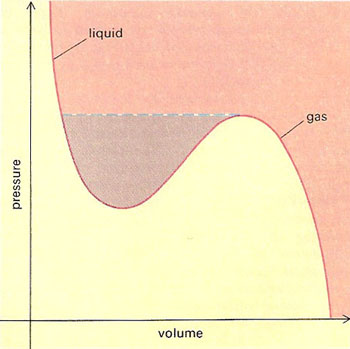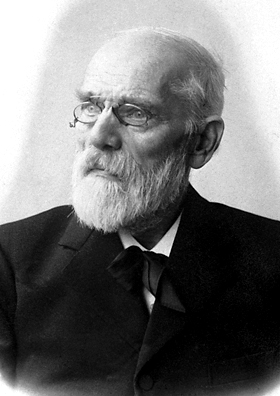A# van der Waals, Johannes Diderick (1837–1923)Graph of the van der Waal's equation for a real gas (red). If a quantity of gas is compressed at constant temperature under normal conditions, the red curve is not followed all the way: at a certain critical pressure a change of state occurs – there is a sudden reduction in volume and the gas liquefies (broken blue line). A whole family of related van der Waals curves exist for different temperatures in three dimensions these give rise to a pressure-volume-temperature surface. For a certain critical temperature, there is no well in the curve, only a point of inflection. At and above this temperature, there is no definable phase transition between the gaseous and liquid states.

Johannes van der Waals was a Dutch physicist who investigated the properties of real gases. Noting that the kinetic theory of gases assumed that the molecules had neither size nor interactive forces between them, in 1873 he proposed the van der Waals' equation of state. The weak attractive force between molecules is called the van der Waals force (see below). He received the 1910 Nobel Prize in Physics.## Van der Waals force

Van der Waals force is an attractive force existing, at very short distances, between atoms and molecules of all substances. The force arises as a result of electrons in neighboring atoms or molecules moving in sympathy with each other. This force is responsible for the term a/v2 in van der Waal's equation of state. In many substances it is small compared with other interatomic attractive and repulsive forces present.

Each atom has a characteristic van der Waals radius: the contact distance between any two atoms is the sum of their van der Waals radii. Two atoms will be attracted to each other by the van der Waals force until the distance between them equals the sum of their van der Waals radii.

## Van der Waals' equation of state

Van der Waals' equation of state is an equation, formulated by Johannes van der Waals, which represents the behavior of ordinary gases more accurately than does the ideal gas law. Van der Waals' equation of state is:

(P + a/V 2)(V - b) = RT

for a gram-molecule of a substance in the gaseous and liquid phases, where P = pressure, V = volume, T = temperature, and R = the gas constant. The term a/V 2 is a correction for the mutual attraction of the molecules, and b is a correction for the actual volume of the molecules themselves. (The values of the constants a and b depend on the particular gas in question.) The attractive forces between molecules are known as van der Waal's forces.